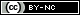## Artigo

•Similares em SciELO

## versão impressa ISSN 1665-2738

#### Resumo

VALDES-PARADA, F. J.; ALVAREZ-RAMIREZ, J  e  OCHOA-TAPIA, J. A.. Analysis of mass transport and reaction problems using green's functions. Rev. Mex. Ing. Quím [online]. 2007, vol.6, n.3, pp.283-294. ISSN 1665-2738.

A methodology to solve typical problems of mass transfer and chemical reaction engineering in terms of Green's functions is presented. The fundamental idea consists on analytically inverting a differential operator by means of the solution of a boundary value problem associated to the original transport and reaction problem. The dependent variable is expressed then as function of the solution of such associated problem, which is the Green's function. Among the advantages that this methodology presents are the smoothing of round-off errors as well as the exact incorporation of boundary conditions. A mandatory requirement for the application of this methodology is that the differential operator must me self-adjoint. To illustrate the potential of the method, diffusion and reaction problems are studied in a catalytic particle involving both linear and non-linear reaction kinetics; in addition, the effect of the external mass transfer resistances is analyzed and the application to non-isothermal, convective, transient and multicomponent problems is discussed. The predictions are compared with those resulting from the numeric solution using finite differences. The analysis is carried out in terms of both numeric (computer time, mesh size) and transport (Thiele modulus, Biot number) parameters.

Palavras-chave : mass transport and reaction; Green's function; finite differences; numeric methods.

· resumo em Espanhol     · texto em Espanhol     · Espanhol (pdf )Todo o conteúdo deste periódico, exceto onde está identificado, está licenciado sob uma Licença Creative Commons